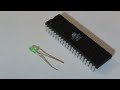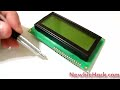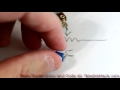### 10 Ohm Through Hole ResistorIntroduction:

A passive device that resists the flow of electricity.

Description:

This resistor will provide 10 Ohms of resistance wherever it is placed and will handle 1/4 watts. Use these low value resistors for voltage dividers and where you need to keep the current flow as high as possible.

10 omh resistor 1/4 watt

\$0.15
Qty:

### Learn about the 10 Ohm Through Hole ResistorVery brief description of a resistor and the resistor's schematic symbol.Physically wiring the circuit for an LED (Light Emitting Diode) to a microcontroller and a resistor so we can turn the LED on and off.Potentiometers are variable resistors. The resistance changes when the wiper is adjusted. The variable resistance is measured from one of the outside leads to the center (wiper) lead. The small vari...The potentiometer is a device that increases or decreases resistance as a variable resistor. Potentiometers can also be used as a voltage divider. If the potentiometer is to be used as a variable res...A voltage divider is a way to decrease a voltage to a proportion of the circuit voltage using two resistors or a potentiometer. The proportion of two resistors will create that proportion output of v... Includes Code